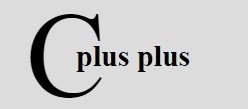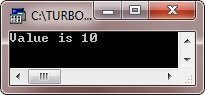# C++ Dynamic Memory

If you want to become an advance C++ programmer, then you must have to achieve good understanding on how to allocates memory at the program runtime. C++ memory is divided into the following two parts:

• heap - unused memory of the program, can be used to allocate the memory, dynamically at the program runtime.
• stack - all the variables declared inside the function will take up the memory from the stack.

### The new operators

Here is the general form to use the new operator to allocate the memory dynamically for any data-type

`new data-type;`

Here, data-type could be any built-in data type.

## C++ Dynamic Memory Example

```/* C++ Dynamic Memory - Example Program */

#include<iostream.h>
#include<conio.h>
void main()
{
clrscr();
int *pval=NULL;     // pointer initialized with NULL
pval=new int;       // request memory for the variable
*pval=10;
cout<<"Value is "<<*pval;
delete pval;
getch();
}```

Here is the sample run of the above C++ program:Let's take one more example program:

```/* C++ Dynamic Memory - Example Program */

#include<iostream.h>
#include<conio.h>
class A
{
public:
A()
{
cout<<"Constructor called\n";
}
~A()
{
cout<<"Destructor called\n";
}
};
void main()
{
clrscr();
A *aob=new A;
delete []aob;
getch();
}```

Below is the sample output of the above C++ program:For more details and more programs on dynamic memory allocation, follow C++ Dynamic Memory Allocation

Tools
Calculator